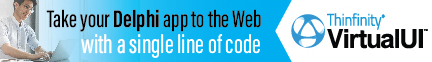DelphiBasicsShowMessageFmtProcedure Display formatted data in a simple dialog with an OK button Dialogs unit
 procedure ShowMessageFmt ( const Formatting : string; const Data : array of const ) ;
Description
The ShowMessageFmt procedure provides 'C' like formatting of multiple of simple data types into a string that is displayed in a single. It provides very precise control over this formatting.

The Formatting parameter defines how the Data array is manipulated into the displayed string.

The dialog has an OK button to allow the user to register the message and close the dialog.

The Formatting string can comprise a mix of ordinary characters (that are passed unchanged to the result string), and data formatting characters. This formatting is best explained by the example code.

In simple terms, each data formatting substring starts with a % and ends with a data type indicator :

 d = Decimal (integer) e = Scientific f = Fixed g = General m = Money n = Number (floating) p = Pointer s = String u = Unsigned decimal x = Hexadecimal

The general format of each formatting substring is as follows:

%[Index:][-][Width][.Precision]Type

where the square brackets refer to optional parameters, and the : . - characters are literals, the first 2 of which are used to identify two of the optional arguments.
Related commands
 InputBox Display a dialog that asks for user text input, with default InputQuery Display a dialog that asks for user text input MessageDlg Displays a message, symbol, and selectable buttons MessageDlgPos Displays a message plus buttons at a given screen position PromptForFileName Shows a dialog allowing the user to select a file ShowMessage Display a string in a simple dialog with an OK button ShowMessagePos Display a string in a simple dialog at a given screen position
Author linksDownload this web site as a Windows program.

 Example code : Showing all of the formatting data types var   text : string; begin  // Just 1 data item   ShowMessageFmt('%s', ['Hello']);   // A mix of literal text and a data item   ShowMessageFmt('String = %s', ['Hello']);   ShowMessage('');   // Examples of each of the data types   ShowMessageFmt('Decimal          = %d', [-123]);   ShowMessageFmt('Exponent         = %e', [12345.678]);   ShowMessageFmt('Fixed            = %f', [12345.678]);   ShowMessageFmt('General          = %g', [12345.678]);   ShowMessageFmt('Number           = %n', [12345.678]);   ShowMessageFmt('Money            = %m', [12345.678]);   ShowMessageFmt('Pointer          = %p', [addr(text)]);   ShowMessageFmt('String           = %s', ['Hello']);   ShowMessageFmt('Unsigned decimal = %u', );   ShowMessageFmt('Hexadecimal      = %x', ); end; Show full unit code Hello   String = Hello      Decimal          = -123   Exponent         = 1.23456780000000E+004   Fixed            = 12345.68   General          = 12345.678   Number           = 12,345,68   Money            = ?12,345.68   Pointer          = 0069FC90   String           = Hello   Unsigned decimal = 123   Hexadecimal      = 8C Example code : Using the index, width and precision values begin  // The width value dictates the output size  // with blank padding to the left  // Note the <> characters are added to show formatting   ShowMessageFmt('Padded decimal    = <%7d>', );   // With the '-' operator, the data is left justified   ShowMessageFmt('Justified decimal = <%-7d>', );   // The precision value forces 0 padding to the desired size   ShowMessageFmt('0 padded decimal  = <%.6d>', );   // A combination of width and precision  // Note that width value precedes the precision value   ShowMessageFmt('Width + precision = <%8.6d>', );   // The index value allows the next value in the data array  // to be changed   ShowMessageFmt('Reposition after 3 strings = %s %s %s %1:s %s',                      ['Zero', 'One', 'Two', 'Three']);   // One or more of the values may be provided by the  // data array itself. Note that testing has shown that an *  // for the width parameter can yield EConvertError.   ShowMessageFmt('In line           = <%10.4d>', );   ShowMessageFmt('Part data driven  = <%*.4d>', [10, 1234]);   ShowMessageFmt('Data driven       = <%*.*d>', [10, 4, 1234]); end; Show full unit code Padded decimal    = <   1234>   Justified decimal = <1234   >   0 padded decimal  = <001234>   Width + precision = <  001234>   Reposition after 3 strings = Zero One Two One Two   In line           = <      1234>   Part data driven  = <      1234>   Data driven       = <      1234>
 Delphi Programming © Neil Moffatt 2002 - 2020. All rights reserved.  |  Home Page